• +91 9971497814
• info@interviewmaterial.com

# RD Chapter 23- The Straight Lines Ex-23.16 Interview Questions Answers

### Related Subjects

Question 1 :
Determine the distance between the following pair of parallel lines:
(i) 4x – 3y – 9 = 0 and 4x – 3y – 24 = 0
(ii) 8x + 15y – 34 = 0 and 8x + 15y + 31 = 0

(i) 4x – 3y – 9 = 0 and 4x– 3y – 24 = 0

Given:

The parallel lines are

4x − 3y − 9= 0 … (1)

4x − 3y − 24= 0 … (2)

Let d be the distancebetween the given lines.

So,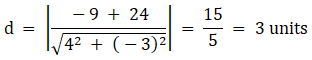The distance betweengivens parallel line is 3units.

(ii) 8x + 15y – 34 = 0 and8x + 15y + 31 = 0

Given:

The parallel lines are

8x +15y − 34 = 0 … (1)

8x + 15y + 31 = 0 …(2)

Let d be the distancebetween the given lines.

So,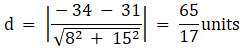The distance betweengivens parallel line is 65/17 units.

Question 2 : Find the equation of two straight lines which are parallel to x + 7y + 2 = 0 and at unit distance from the point (1, -1).

Given:

The equation is parallelto x + 7y + 2 = 0 and at unit distance from the point (1, -1)

The equation of givenline is

x + 7y + 2 = 0 … (1)

The equation of a lineparallel to line x + 7y + 2 = 0 is given below:

x + 7y + λ =0 … (2)

The line x + 7y+ λ = 0 is at a unit distance from the point (1, − 1).

So,

1 =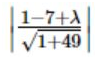λ – 6 = ± 5√2

λ = 6 + 5√2, 6 – 5√2

now, substitute thevalue of λ back in equation x + 7y + λ = 0, we get

x + 7y + 6 + 5√2 = 0 and x + 7y + 6 – 5√2

The required lines:

x + 7y + 6 + 5√2 = 0 and x + 7y + 6 – 5√2

Question 3 : Prove that the lines 2x + 3y = 19 and 2x + 3y + 7 = 0 are equidistant from the line 2x + 3y = 6.

Given:

The lines A, 2x + 3y =19 and B, 2x + 3y + 7 = 0 also a line C, 2x + 3y = 6.

Let d1 bethe distance between lines 2x + 3y = 19 and 2x + 3y = 6,

While d2 isthe distance between lines 2x + 3y + 7 = 0 and 2x + 3y = 6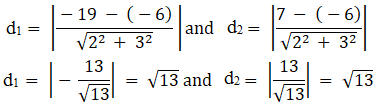Hence proved, thelines 2x + 3y = 19 and 2x + 3y + 7 = 0 are equidistant from the line 2x + 3y =6

Question 4 : Find the equation of the line mid-way between the parallel lines 9x + 6y – 7 = 0 and 3x + 2y + 6 = 0.

Given:

9x + 6y – 7 = 0 and 3x+ 2y + 6 = 0 are parallel lines

The given equations ofthe lines can be written as:

3x + 2y – 7/3 =0 … (1)

3x + 2y + 6 = 0 … (2)

Let the equation ofthe line midway between the parallel lines (1) and (2) be

3x + 2y+ λ = 0 … (3)

The distance between(1) and (3) and the distance between (2) and (3) are equal.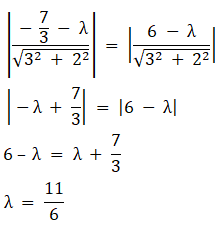Now substitute thevalue of λ back in equation 3x + 2y + λ = 0, we get

3x + 2y+ 11/6 = 0

By taking LCM

18x + 12y + 11 = 0

The required equationof line is 18x + 12y + 11 = 0

Todays Deals### RD Chapter 23- The Straight Lines Ex-23.16 Contributorskrishan

Name:
Email:

# Latest News# 9000 interview questions in different categories